# List all Worksheet Names

This post will guide you how to get a list of all worksheet names in an excel workbook. How do I List the Sheet names with Formula in Excel. How to generate a list of all sheet tab names using Excel VBA Code.

Assuming that you have a workbook that has hundreds of worksheets and you want to get a list of all the worksheet names in the current workbook. And the below will introduce 3 methods with you.

## Get All Worksheet Names Manually

If there are only few worksheets in your workbook, and you can get a list of all worksheet tab names by manually. Let’s see the below steps:

#2 double click on the sheet’s name in the sheet tab. Press Ctrl + C shortcuts in your keyboard to copy the selected sheet.

#3 create a notepad file, and then press Ctrl +V to paste the sheet name.

#4 follow the above steps 2-3 to copy&paste all worksheet names into notepad file.

## Get All Worksheet Names with Formula

You can also use a formula to get a list of all worksheet names with a formula. You can create a formula based on the LOOKUP function, the CHOOSE function, the INDEX function, the MID function, the FIND function and the ROWS function. Just do the following steps:

#1 go to FORMULAS tab, click Name Manager command under Defined Names group. The Name Manager dialog will open.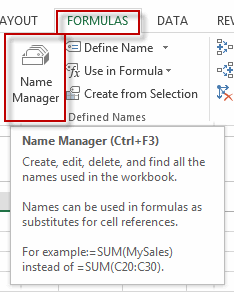#2 click New… button to create a define name, type Sheets in the Name text box, and type the formula into the Refers to text box.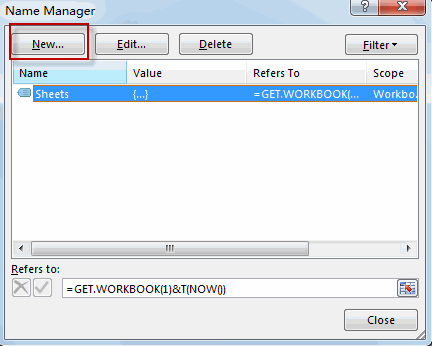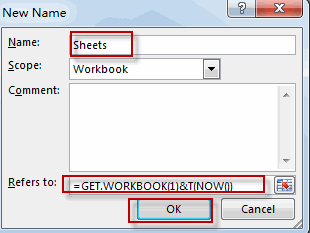`=GET.WORKBOOK(1)&T(NOW())`

#3 Type the following formula into a blank cell and press Enter key in your keyboard, and then drag the autofill handle over others cells to get the rest sheet names.

`=LOOKUP("xxxxx",CHOOSE({1,2},"",INDEX(MID(Sheets,FIND("]",Sheets)+1,255),ROWS(A\$1:A1))))`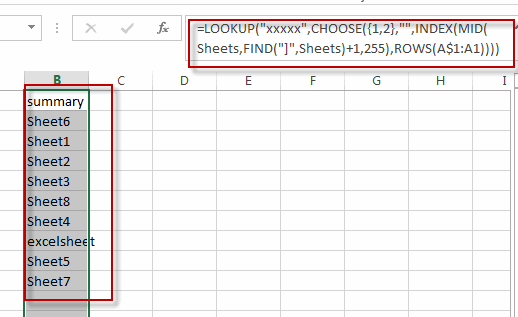You will see that all sheet names have been listed in the cells.

## Get All Sheet Names with Excel VBA Macro

You can also use an Excel VBA Macro to quickly get a list of all worksheet tab names in your workbook. Just do the following steps:

#1 open your excel workbook and then click on “Visual Basic” command under DEVELOPER Tab, or just press “ALT+F11” shortcut.#2 then the “Visual Basic Editor” window will appear.

#3 click “Insert” ->”Module” to create a new module#4 paste the below VBA code into the code window. Then clicking “Save” button.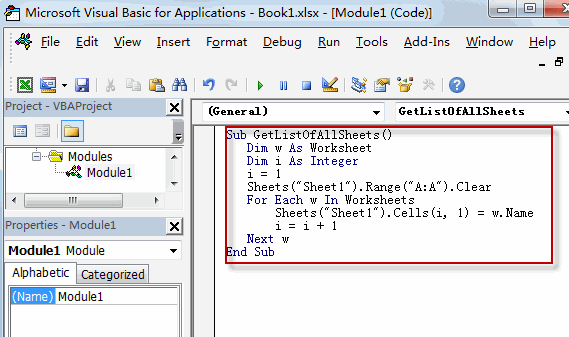```Sub GetListOfAllSheets()
Dim w As Worksheet
Dim i As Integer
i = 1
Sheets("Sheet1").Range("A:A").Clear
For Each w In Worksheets
Sheets("Sheet1").Cells(i, 1) = w.Name
i = i + 1
Next w
End Sub```

#5 back to the current worksheet, then run the above excel macro. Click Run button.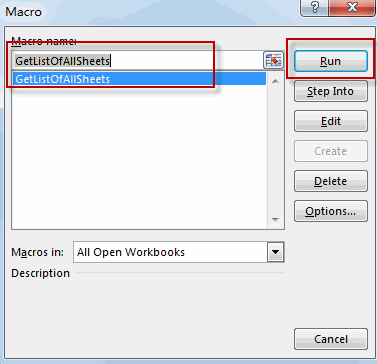#6 Let’s see the result.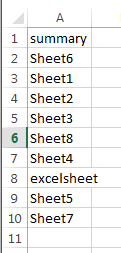### Related Functions

• Excel MID function
The Excel MID function returns a substring from a text string at the position that you specify.The syntax of the MID function is as below:= MID (text, start_num, num_chars)…
• Excel LOOKUP function
The Excel LOOKUP function will search a value in a vector or array.The LOOKUP function is a build-in function in Microsoft Excel and it is categorized as a Lookup and Reference Function.The syntax of the LOOKUP function is as below:= LOOKUP (lookup_value, lookup_vector, [result_vector])…
• Excel Choose Function
The Excel CHOOSE function returns a value from a list of values. The CHOOSE function is a build-in function in Microsoft Excel and it is categorized as a Lookup and Reference Function.The syntax of the CHOOSE function is as below:=CHOOSE (index_num, value1,[value2],…)…
• Excel INDEX function
The Excel INDEX function returns a value from a table based on the index (row number and column number)The INDEX function is a build-in function in Microsoft Excel and it is categorized as a Lookup and Reference Function.The syntax of the INDEX function is as below:= INDEX (array, row_num,[column_num])…
• Excel ROWS function
The Excel ROWS function returns the number of rows in a cell reference.The syntax of the ROWS function is as below:= ROWS(array)…
• Excel FIND function
The Excel FIND function returns the position of the first text string (sub string) within another text string.The syntax of the FIND function is as below:= FIND(find_text, within_text,[start_num])
Related Posts

Find and Replace Multiple Values

This post will guide you how to find and replace multiple values at once with VBA macro or using formula  in Excel. How do I make multiple find and replace in Excel. Suppose that you have a few cells containing ...

VBA Macro For VLOOKUP From Another Sheet

In the previous post, you should know that how to fix or remove the #N/A error when using VLOOKUP formula to lookup value from another sheet. And this post will show you how to use VBA code to vlookup data ...

How To Insert Comments in Protected Worksheet in Excel

This post will show you how to allow comments in a protected worksheet in Excel. You can easily to insert comments into cells in a normal worksheet in Excel, but if want to insert a comment in a worksheet that ...

How To Convert Text to Upper Cases(Using VBA) in Excel

This post will show you how to switch from lower case to upper case in Excel. and I am going to show you two different ways of converting text to upper cases using formula or VBA macro in Excel 2013,Excel ...

How To Hide Every Other Row in Excel (Using VBA)

This post will show you how to hide alternate rows or columns in Excel or how to hide every third, fourth, fifth row or column in Excel. If you want to hide every other row in your current worksheet, how ...

Sidebar